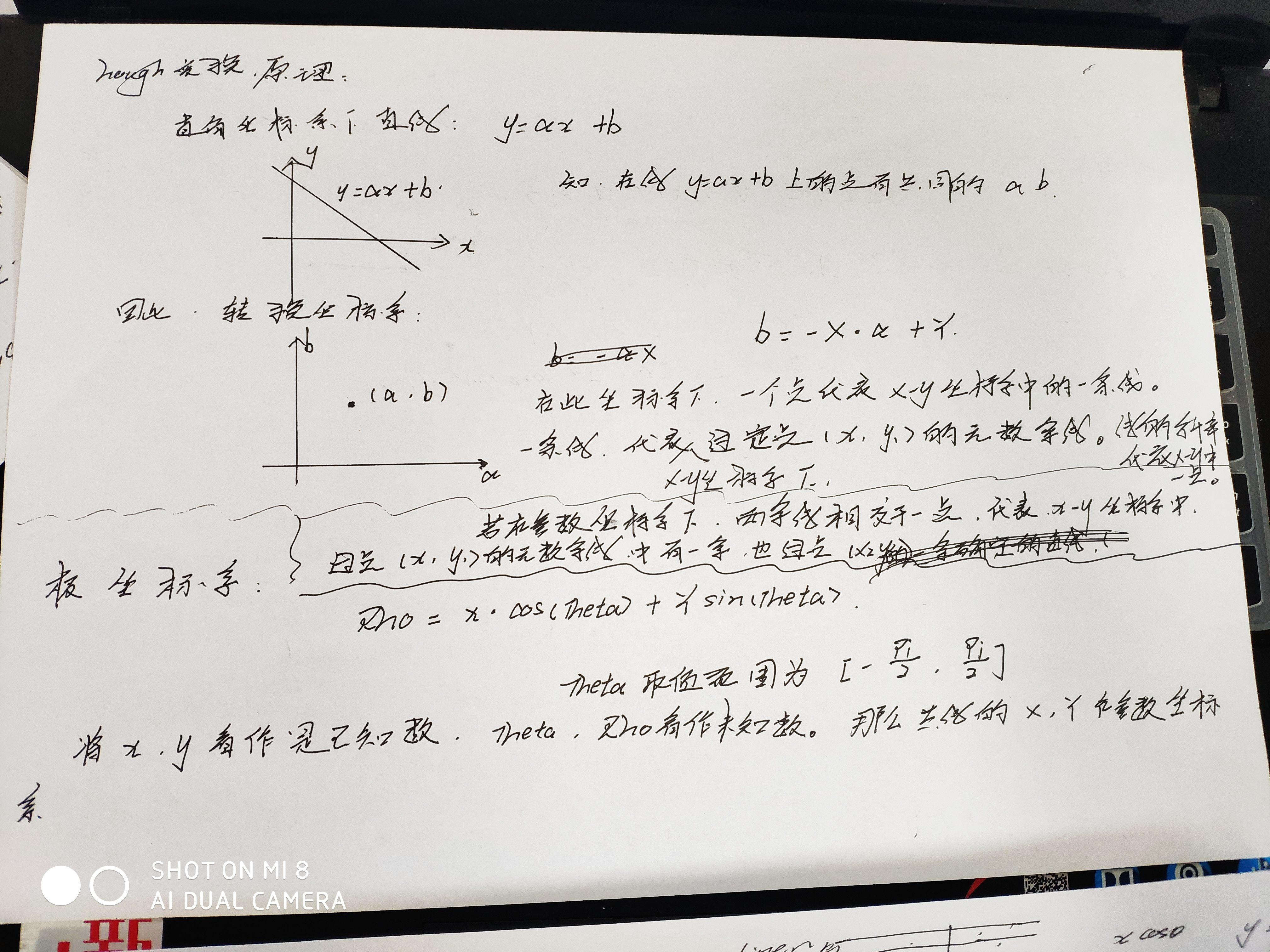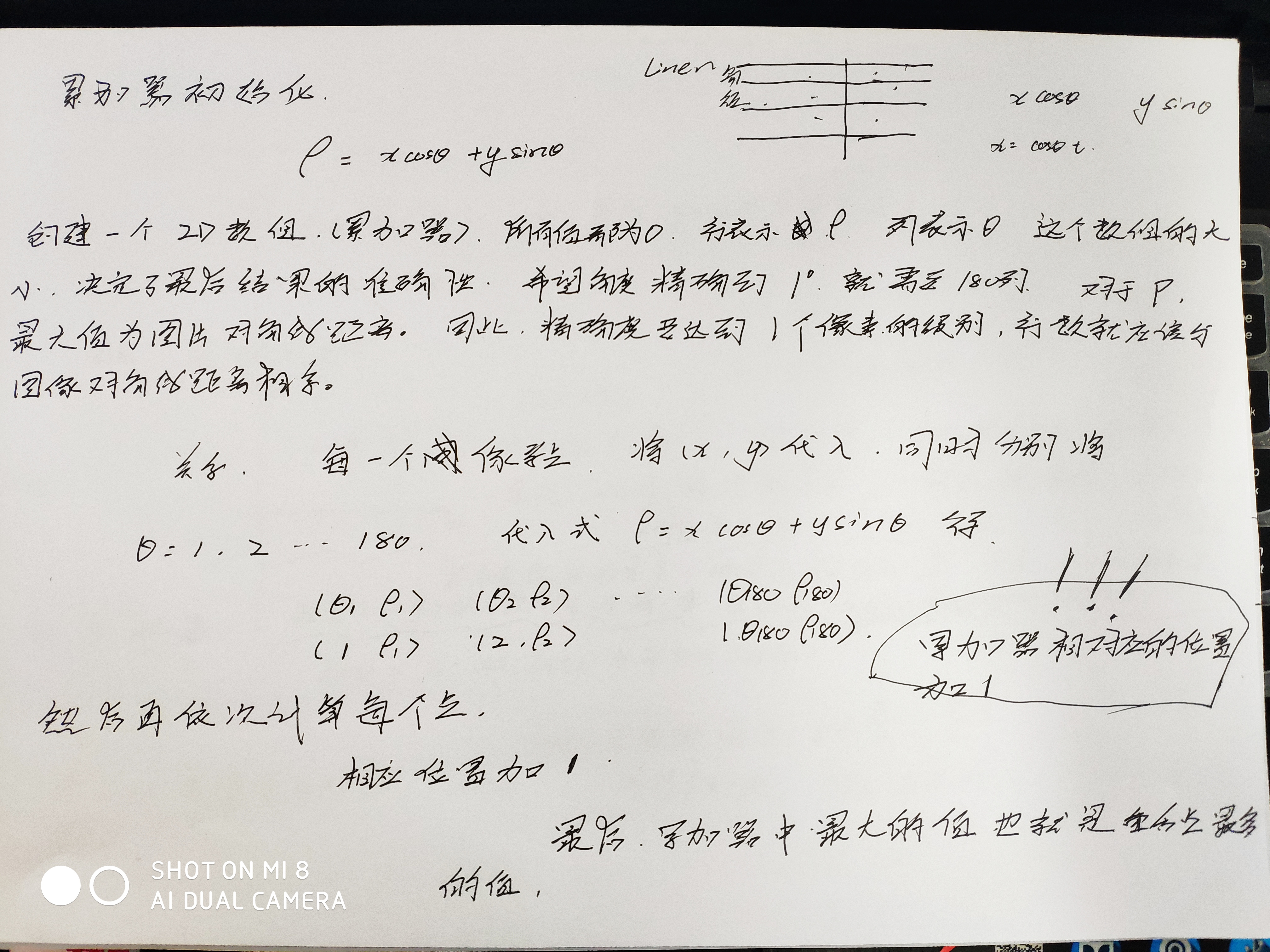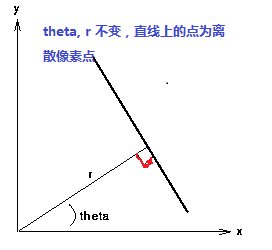2017-01-20 10:07:54 yangdashi888 阅读数 944

1、霍夫变换可以用于检测直线和圆，其主要是利用了极坐标的方式。

X *cos(theta) + y * sin(theta)  = r 其中角度theta指r与X轴之间的夹角，r为到直线几何垂(x –a ) ^2 + (y-b) ^ 2 = r^2其中(a, b)为圆的中心点坐标，r圆的半径。这样霍夫的参数空间就

2019-03-09 14:31:21 qq_37855507 阅读数 162

## 一、直线检测：

### 1.直角坐标参数空间：``````#include<opencv2\opencv.hpp>
#include<stdio.h>
#include<iostream>

using namespace cv;
using namespace std;

int main(int arg, char* argv[])
{
//在如图片并显示；
//namedWindow("原图");
//imshow("原图", img);

destroyAllWindows();
//将图像灰度化并显示
Mat grayImage; //创建无初始化矩阵
cvtColor(img, grayImage, CV_RGB2GRAY);
//namedWindow("灰度图");
//imshow("灰度图", grayImage);
//边缘检测
Mat edge;
Canny(grayImage, edge, 3, 9, 3);

int i, j;

//行列
int row = grayImage.rows;
int col = grayImage.cols;

//极径最大值为对角线+宽
//cvCeil 取整，返回不小于参数的整数。
int max_r = col + cvCeil(sqrt(double(row*row + col*col)));

//累加器 三角函数
int *line_cnt;  //指针数组，  是一个普通的数组，数组中的每一个元素都是指针。
double sin_, cos_, rad_ = CV_PI / 180;
for (i = 0; i < 180; i++)
{
//初始化累加器为0
line_cnt[i] = new int[max_r]();   //列为180列，列表示theta，行表示r,r最大值为max_r;
//初始化三角函数
}

//极径，极角
int r = 0;
int theta = 0;

//遍历图像，判断并进行累加。
uchar *p;
for (i = 0; i < row; i++)
{
//用指针遍历图像中的元素。
//每一行图像的指针；
p = edge.ptr<uchar>(i);
for (j = 0; j < col; j++)
{
if (p[j] != 0)
{
//不等于0，证明是有用的边缘像素点
//由该点的参数方程 更改累加器
for (theta = 0; theta < 180; theta++)
{
//极坐标 直线方程
r = cvRound(j*cos_[theta] + i*sin_[theta]);
//偏移量， 因为累计器（霍夫矩阵的索引没有负值）
r = r + col;
//累加器完成累加
line_cnt[theta][r]++;
}
}
}
}

//存放取出最长的n条线
int n = 6;
int *line_n;            //三行分别用来存储极角、极径、共点数目
line_n = new int[n]();  //极角
line_n = new int[n]();  //极径
line_n = new int[n]();  //共点数目

int tt = 0, rr = 0, cnt = 0;
//寻找累加器中最大的值
for (theta = 0; theta < 180; theta++)
{
for (r = 0; r < max_r; r++)
{
//最少共点 < 这条直线的共点 则替换 并尝试进行冒泡
if (line_n[n-1] < line_cnt[theta][r])
{
line_n[n-1] = theta;
//累计的时候偏移过，将偏移修改回来
line_n[n-1] = r - col;
line_n[n-1] = line_cnt[theta][r];
//冒泡排序
for (i = n - 1; i > 0; i--)
{
//如果大于 则交换
if (line_n[i] > line_n[i - 1])
{
tt = line_n[i];
rr = line_n[i];
cnt = line_n[i];
line_n[i] = line_n[i - 1];
line_n[i] = line_n[i - 1];
line_n[i] = line_n[i - 1];
line_n[i - 1] = tt;
line_n[i - 1] = rr;
line_n[i - 1] = cnt;
}
else
break;
}
}
}
}

//画出线段
for (i = 0; i < n; i++)
{
Point pt1, pt2;
double a = cos_[line_n[i]], b = sin_[line_n[i]];
double x0 = a*line_n[i], y0 = b*line_n[i];
//用点画出检测到的直线。
//下面四个式子为纯直线几何推导。
pt1.x = cvRound(x0 + max_r*(-b));
pt1.y = cvRound(y0 + max_r*(a));
pt2.x = cvRound(x0 - max_r*(-b));
pt2.y = cvRound(y0 - max_r*(a));

//绿线
line(img, pt1, pt2, Scalar(0, 255, 0), 1, CV_AA);
}
cvNamedWindow("直线检测图");
imshow("直线检测图",img);
waitKey(0);
//----------------------释放内存-----------------
for (i = 0; i < 180; i++)
{
delete[]line_cnt[i];
}
delete[]line_n;
delete[]line_n;
delete[]line_n;
return 0;
}
``````

2018-09-11 11:00:34 qq_18234121 阅读数 414

### 原理介绍让我们来看看霍夫变换是如何工作的。每一条直线都可以用(ρ; θ) 表示。所以首先创建一个2D 数组（累加器），初始化累加器，所有的值都为0。行表示ρ，列表示θ。这个数组的大小决定了最后结果的准确性。如果你希望角度精确到1 度，你就需要180 列。对于ρ，最大值为图片对角线的距离。所以如果精确度要达到一个像素的级别，行数就应该与图像对角线的距离相等。

想象一下我们有一个大小为100x100 的直线位于图像的中央。取直线上的第一个点，我们知道此处的（x，y）值。把x 和y 带入上边的方程组，然后遍历θ 的取值：0，1，2，3，...，180。分别求出与其对应的 的值，这样我们就得到一系列(ρ; θ) 的数值对，如果这个数值对在累加器中也存在相应的位置，就在这个位置上加1。所以现在累加器中的（50，90）=1。（一个点可能存在与多条直线中，所以对于直线上的每一个点可能是累加器中的多个值同时加1）。

### 编程思路解析：

1.      读取一幅带处理二值图像，最好背景为黑色。

2.      取得源像素数据

3.      根据直线的霍夫变换公式完成霍夫变换，预览霍夫空间结果

4.       寻找最大霍夫值，设置阈值，反变换到图像RGB值空间(程序难点之一)

5.      越界处理，显示霍夫变换处理以后的图像

2017-07-21 19:45:58 weixiankui1997 阅读数 1289

程序输入的是一段视频，拍摄的是一条白色直线。

```vector <Vec2f> lines;//the rho and theta of each line
HoughLines(edge,lines,0.1,CV_PI/360,13,0,0);```

```sort(arr_rho,arr_rho+lines.size());
//for(int i=(total-count);i<total;i++)//because the heading (total-count) values in the array is zero
//   cout<<"rho"<<arr_rho[i]<<endl;
double rho_mid_l,rho_mid_r;
static int divide;
double count_l=0,count_r=0;
bool left=1;
for(int i=(total-count);i<total;i++)
{
if((abs(arr_rho[i]-arr_rho[i+1]) > 10*abs(arr_rho[i+1]-arr_rho[i+2])));
{
left = 0;
divide = i;
}
if(left)
{
rho_mid_l += arr_rho[i];
count_l++;
}
else
{
rho_mid_r += arr_rho[i];
count_r++;
}
}
rho_mid_l += arr_rho[divide];
count_l++;
rho_mid_r -= arr_rho[divide];
count_r--;
rho_mid_l = rho_mid_l/count_l;
rho_mid_r = rho_mid_r/count_r;
rho_mid=(rho_mid_l+rho_mid_r)/2.0;```

`if((abs(arr_rho[i]-arr_rho[i+1]) > 10*abs(arr_rho[i+1]-arr_rho[i+2]))&&left&&(abs(arr_rho[i+1]-arr_rho[i])>10))`
```&&left 就防止了divide的值不正确的情况（考虑arr_rho[]={100,101,200,200.9,201，201.1}，这种情况下divede就会是3，而正确的应该是2）
&&(abs(arr_rho[i+1]-arr_rho[i])>10)) 则避免的这种情况下的误判：arr_rho[]={100,102,100.01,201,202,} 若没有这个条件程序就会判断100后面的rho都是另外一边的了

* video.cpp
* Author:
*   Weixiankui
*/
#include <iostream> // for standard I/O
#include <string>   // for strings
#include <iomanip>  // for controlling float print precision
#include <sstream>  // string to number conversion
#include <opencv2/core.hpp>     // Basic OpenCV structures (cv::Mat, Scalar)
#include <opencv2/imgproc.hpp>  // Gaussian Blur
#include <opencv2/videoio.hpp>
#include <opencv2/highgui.hpp>

using namespace std;
using namespace cv;

double Distance(Point core,Point pt1,Point pt2)
{
double A,B,C,dis;
A=pt2.y-pt1.y;
B=pt1.x-pt2.x;
C=pt2.x*pt1.y-pt1.x*pt2.y;
dis=abs(A*core.x+B*core.y+C)/sqrt(A*A+B*B);
return dis;
}

void MiddleLine(Mat image,Point *points);

int main(int argc,char **argv)
{
if(argc!=2)
{
printf("usage:\n./video <path of video>\n");
return -1;
}
const char *win="video~";
VideoCapture video(argv);
namedWindow(win,WINDOW_AUTOSIZE);
char key=0;

for(;;)
{

Mat src;
video>>src;
Point points;
MiddleLine(src,points);//input the image and get two points of the middle line int the Point array(2nd parament)
line(src,points,points,Scalar(0,0,255),2,CV_AA);//draw the line
imshow(win,src);
double dist = Distance(core,points,points);//get the distance between the middle line and the center point
cout<<"Distance"<<dist<<endl;
waitKey(1);

}
return 0;
}

void MiddleLine(Mat image ,Point *points)
{
//Point points;//the two point of the midline to be return;
Mat src = image.clone();
int blur_value=5;//modify the blur_value to make the ebge(you can use imshow("edge",edge) to see it) clear
Mat edge,dst;

medianBlur(src,src,blur_value+2);
blur(src,src,Size(blur_value,blur_value));

Canny(src,edge,20,75,3);
cvtColor(edge,dst,CV_GRAY2BGR);
//imshow("edge",edge);
vector <Vec2f> lines;
HoughLines(edge,lines,0.1,CV_PI/360,13,0,0);
//cout<<"lines.size()  "<<lines.size()<<endl;
float theta_mid=0,rho_mid=0;
int count=0,total=0;
float arr_rho[lines.size()];
for(size_t i=0;i<lines.size();i++)//
arr_rho[i]=0;
float sum;
//for(size_t i=0;i<lines.size();i++)
//{
//    cout<<"rho  "<<lines[i]<<"  theta  "<<lines[i]<<endl;
//}
//cout<<"------------------------"<<endl;
for(size_t i=0;i<lines.size();i++)//
{
total ++;
if(abs(lines[i]-CV_PI/2.0)<0.5) continue;//
//float rho=lines[i],theta = lines[i];
theta_mid +=lines[i]>0?lines[i]:(lines[i]-CV_PI);

arr_rho[count]=abs(lines[i]);
sum += lines[i]*0.1;
//cout<<"count"<<count<<endl;
//cout<<"rho"<<arr_rho[count]<<endl;
count++;

}
sort(arr_rho,arr_rho+lines.size());
//for(int i=(total-count);i<total;i++)//because the heading (total-count) values in the array is zero
//   cout<<"rho"<<arr_rho[i]<<endl;
double rho_mid_l,rho_mid_r;
static int divide;
double count_l=0,count_r=0;
bool left=1;
for(int i=(total-count);i<total;i++)
{
if((abs(arr_rho[i]-arr_rho[i+1]) > 10*abs(arr_rho[i+1]-arr_rho[i+2]))&&left&&(abs(arr_rho[i+1]-arr_rho[i])>10))//add the && left condition other wise the divide may be given to numer,and the program would have problem;
{
left = 0;
divide = i;
//        cout<<"divide"<<divide<<endl;
}
if(left)
{
rho_mid_l += arr_rho[i];
count_l++;
}
else
{
rho_mid_r += arr_rho[i];
count_r++;
}
//    cout <<"count_l&count_r"<<count_l<<count_r<<endl;
}
rho_mid_l += arr_rho[divide];
count_l++;
rho_mid_r -= arr_rho[divide];
count_r--;
//cout<<"divide"<<divide<<endl<<"count_l&count_r"<<count_l  << count_r<<endl;
rho_mid_l = rho_mid_l/count_l;
//cout << "rho_mid_l" << rho_mid_l<<endl;
rho_mid_r = rho_mid_r/count_r;
//cout << "rho_mid_r"<<rho_mid_r<<endl;
rho_mid=(rho_mid_l+rho_mid_r)/2.0;
float rho;
if(sum>0)
rho=rho_mid;
else
rho=-rho_mid;
rho=theta_mid>0?rho_mid:-rho_mid;
//theta=theta_mid
theta_mid=theta_mid/count;
float theta=theta_mid>0?theta_mid:(CV_PI+theta_mid);
Point pt1,pt2;
double a =cos(theta),b=sin(theta);
double x0=a*rho,y0=b*rho;

points.x=cvRound(x0+10000*(b));
points.y=cvRound(y0+10000*(-a));
points.x=cvRound(x0+10000*(-b));
points.y=cvRound(y0+10000*(a));
cout<<"theta"<<theta<<endl;
//cout<<"~~~~~~~~~~~~~~~~~~~~~"<<endl;
//return points;
}

```

2016-03-24 15:08:44 qqh19910525 阅读数 424